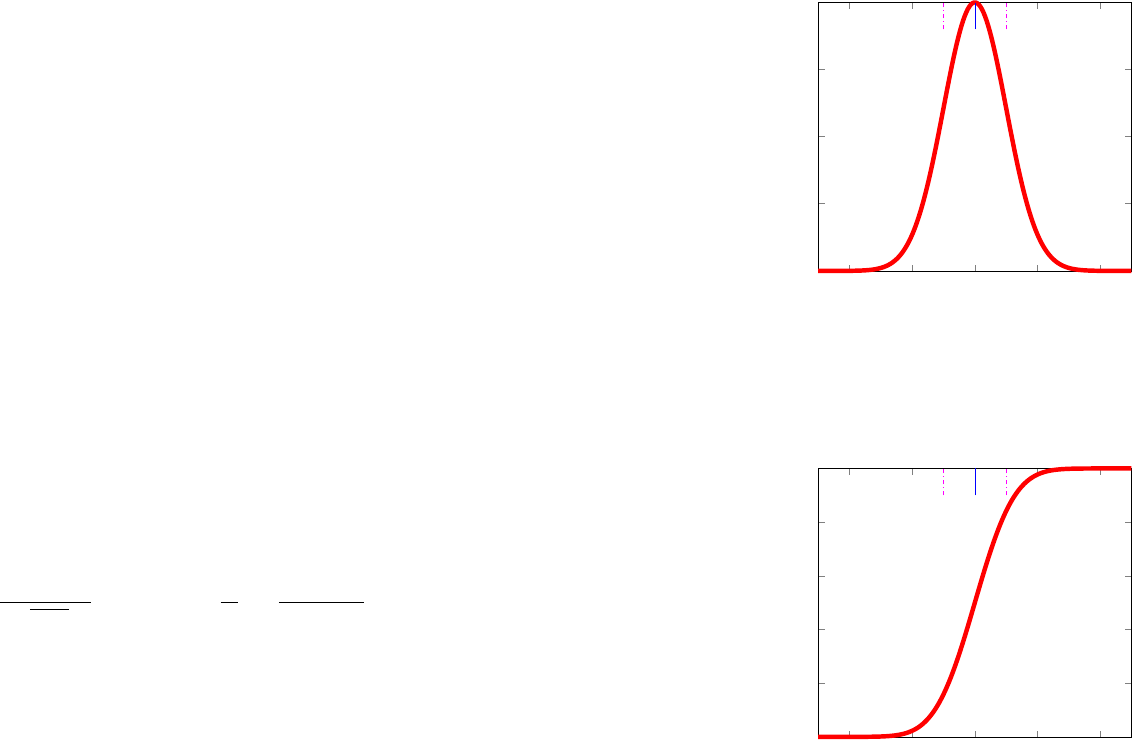4
Probability Distributions
The deﬁnition of probability distributions
f
X
(
x
) was left aside in
chapter 3. This chapter presents the formulation and properties for
the probability distributions employed in this book: the Normal
distribution for
x 2 R
, the log-normal for
x 2 R
+
, and the Beta for
x 2 (0, 1).
4.1 Normal Distribution
The most widely employed probability distribution is the Normal,
also known as the Gaussian, distribution. In this book, the names
Gaussian and Normal are emp loyed interchangeably when describ-
ing a probability distribu t ion . This section covers the math em at ic al
foundation for the univariate and multivariate Normal and then
details the properties explaining its widespread usage.
4.1.1 Univariate Normal
Univariate Normal
x 2 R : X N(x; µ,
2
)
4 2 0 2 4
0
0.1
0.2
0.3
µ
µ +
µ
x
f
X
(x)
(a) Probability density function (PDF)
4 2 0 2 4
0
0.2
0.4
0.6
0.8
1
µ
µ +
µ
x
F
X
(x)
(b) Cumulative distribution function (CDF)
Figure 4.1: Representation of the univari-
ate Normal for µ =0, =1.
The probability density function (PDF) for a Normal random
variable is deﬁned over the real numbers
x 2 R
.
X N
(
x
;
µ,
2
)is
parameterized by its me